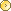Visitors Online: 77 | Monday 23rd September 2019

CBSE Guess > Papers > Question Papers > Class X > 2005 > Science > Delhi Set-II

SCIENCE AND TECHNOLOGY—2005 (Set II—Outside Delhi)

Except for the following questions, all the remaining questions have been asked in Set 1.

SECTION A

Q. 1. A wire of resistance 5 ohm is bent in the form of a closed circle. What is the effective resistance between the two points at the ends of any diameter of the circle? 1

Q. 3. Write the chemical equation to represent the reaction taking place between sodium metal and cold water. 1

Q. 7. Light enters from air into water which has a refractive index of 1.33. Calculate the speed of light in water. The speed of light in air is 3.0 x 108 ms -1 2

Q. 9. What is observed when some drops of conc. H2SO4 are added to sugar (C12H22O11) placed in a test tube? Write the chemical equation to represent the reaction taking place. 2

Q. 13. If a 24 V battery is connected to the arrangement of resistances given below,
calculate: 3
the total effective resistance of the circuit and
the total current flowing in the circuit.Q. 16. Draw a labelled diagram of the Frasch process for extracting sulphur from a deep underground mine. 3

Q. 18. Draw the lines of force (indicating field direction) of the magnetic field through and around (a) a single loop of wire carrying electric current, and (b) a solenoid carrying electric current. 5

SECTION B

Q. 22. What is 'retrovirus'? 1

Q. 25. When is water considered to be polluted? Write two harmful effects of using polluted water. 2

Q. 30. Draw a diagram of the 'human alimentary canal'. Label the following body parts on it: 5
(i) Liver (ii) Pancreas
(iii) Gallbladder (Iv) Duodenum

Write one function each of the liver and the pancreas.

Science 2005 Question Papers Class X

 CBSE 2005 Question Papers Class XEnglishHindiScienceMathematicsSocialStudiesSanskritFrenchAdditional Subjets